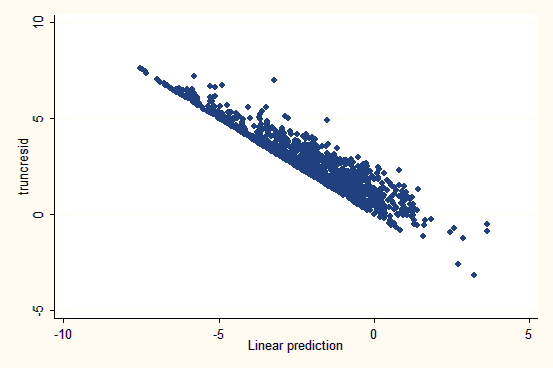[Date Prev][Date Next][Thread Prev][Thread Next][Date index][Thread index]

# st: normality and heteroskedasticity in truncreg

 From Anita To statalist@hsphsun2.harvard.edu Subject st: normality and heteroskedasticity in truncreg Date Wed, 26 Mar 2008 08:32:42 +0100

```Dear Statalist Members
```

I am estimating a truncated regression with truncreg. My y-Variable is truncated (400 of 1700 observations) and highly skewed to the right, so I transformed using the logarithm.
Now I would like to test for Heteroskedasticity and Normality. I did not find any tests.

I tried to analize the residuals graphically by

predict, xb
gen residual=y-xb, where y ist my observation and xb is the fitted value.

By plotting the residuals against the fitted values, I found a graph with a slope of -1, above this, there are also some observations (graph ist attached).
How can I interpret this, or is there a better way to interpret truncated regression residuals for heteroscedasticity? Why are there so many negative fitted values? May be I made a mistake calculating the residuals

I also tried to follow the graphical analysis for normal regression for Normality using qnorm and pnorm, but i am not shure if this is appropriate, as this is not a linear estimation.

Can anyone help me please?
I am very sorry, if this is a silly question

Anita© Copyright 1996–2019 StataCorp LLC   |   Terms of use   |   Privacy   |   Contact us   |   What's new   |   Site index